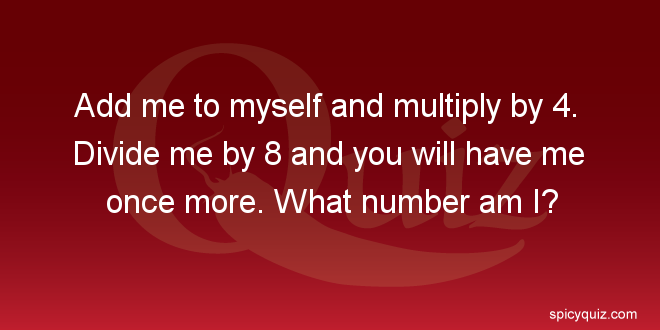Monday , September 28 2020
Home / Math / Find the right number in this math riddle# Find the right number in this math riddle

Add me to myself and multiply by 4. Divide me by 8 and you will have me once more. What number am I?

### Hint

Try different numbers to go to the right answer.

Any number.

## How many squares do you see?

How many squares do you see? This is the question that you must give an …

### One comment

1.any number works, duh